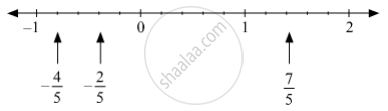SSC (English Medium) Class 8Maharashtra State Board
Share

# Show the Following Numbers on a Number Line. Draw a Separate Number Line for Each Example. 7 5 , − 2 5 , − 4 5 - SSC (English Medium) Class 8 - Mathematics

ConceptTo Show Rational Numbers on a Number Line

#### Question

Show the following numbers on a number line. Draw a separate number line for each example.

7/5 , (-2)/5 , (-4)/5

#### Solution

7/5 , -2/5 , -4/5 can be represented on the number line as follows.Is there an error in this question or solution?

#### APPEARS IN

Balbharati Solution for Balbharati Class 8 Mathematics (2019 to Current)
Chapter 1: Rational and Irrational numbers
Practice Set 1.1 | Q: 1.2 | Page no. 2
Solution Show the Following Numbers on a Number Line. Draw a Separate Number Line for Each Example. 7 5 , − 2 5 , − 4 5 Concept: To Show Rational Numbers on a Number Line.
S